Definition: probability distribution from The Penguin Dictionary of Science

The distribution of the values of a ➤random variable given either by listing the probabilities (discrete case) or by specifying the probability density function (continuous case).

Summary Article: III.71 Probability Distributions
from The Princeton Companion to Mathematics
1 Discrete Distributions

When we toss a coin, we have no idea whether it will land heads or tails. However, there is a different sense in which the behavior of the coin is highly predictable: if it is tossed many times, then the proportion of heads is very likely to be close to ½.

In order to study this phenomenon mathematically, we need to model it, and this is done by defining a sample space, which represents the set of possible outcomes, and a probability distribution on that space, which tells you their probabilities. In the case of a coin, the natural sample space is the set {H, T}, and the obvious distribution assigns the number ½ to each element. Alternatively, since we are interested in the number of heads, we could use the set {0, 1} instead: after one toss, there is a probability of ½ that the number of heads is 0 and a probability of ½ that it is 1. More generally, a (discrete) sample space is simply a set Ω, and a probability distribution on Ω is a way of assigning a nonnegative real number to each element of Ω in such a way that the sum of all these numbers is 1. The number assigned to a particular element of Ω is then interpreted as the probability that some corresponding outcome will occur, the total probability being 1.

If Ω is a set of size n, then the uniform distribution on Ω is the probability distribution that assigns a probability of 1/n to each element of Ω. However, it is often more appropriate to assign different probabilities to different outcomes. For example, given any real number p between 0 and 1, the Bernoulli distribution with parameter p on the set {0, 1} is the distribution that assigns the number p to 1 and 1 – p to 0. This can be used to model the toss of a biased coin.

Suppose now that we toss an unbiased coin n times. If we are interested in the outcome of every toss, then we would choose the sample space consisting of all possible sequences of 0s and 1s of length n. For instance, if n = 5, a typical element of the sample space is 01101. (This particular element represents the outcome tails, heads, heads, tails, heads, in that order.) Since there are 2n such sequences and they are all equally likely, the appropriate distribution on this space will be the uniform one, which assigns a probability of 1/2n to each sequence.

But what if we are interested not in the particular sequence of heads and tails but just in the total number of heads? In that case, we could take as our sample space the set {0, 1, 2, . . ., n}. The probability that the total number of heads is k is 2n times the number of sequences of 0s and 1s that contain exactly k 1s. This number is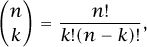so the probability we assign to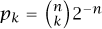.

More generally, for a sequence of n independent experiments, each with the same probability p of success, the probability of a given sequence of k successes and nk failures is pk(1 − p)n–k. So, the probability of having exactly k successes is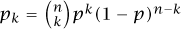. This is called the binomial distribution with parameters n and p. It models the number of heads if you toss a biased coin n times, for example.

Suppose we perform such experiments for as long as we need to in order to obtain one success. When k experiments are performed, the probability of getting k−1 failures followed by a success is pk = (1 − p)k–1 p. Therefore, this formula gives us the distribution of the number of experiments up to the first success. It is called the geometric distribution of parameter p. In particular, the number of tosses of a fair coin needed to get the first head has a geometric distribution of parameter ½ . Notice that our sample space is now the set of all nonnegative integers—in particular, it is infinite. So in this case the condition that the probabilities add up to 1 is requiring that a certain infinite series (the series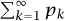) converges to 1.

Now let us imagine a somewhat more complicated experiment. Suppose we have a radioactive source that occasionally emits an alpha particle. It is often reasonable to suppose that these emissions are independent and equally likely to occur at any time. If the average number of emissions per minute is λ, say, then what is the probability that during any given minute there will be k particles emitted?

One way to think about this question is to divide up the minute into n equal intervals, for some large n. If n is large enough, then the probability of two emissions occurring in the same interval is so small that it can be ignored, and therefore, since the average number of emissions per minute is λ, the probability of an emission during any given interval must be approximately λ/n. Let us call this number p. Since the emissions are independent, we can now regard the number of emissions as the number of successes when we do n trials, each with probability p of success. That is, we have the binomial distribution with parameters n and p, where p = λ/n.

Notice that as n gets larger, p gets smaller. Also, the approximations just made become better and better. It is therefore natural to let n tend to infinity and study the resulting “limiting distribution.” It can be checked that, in the limit as n, the binomial probabilities converge to pk = eλλk/k!. These numbers define a distribution on the set of all nonnegative integers, known as the Poisson distribution of parameter λ.

2 Probability Spaces

Suppose that I throw a dart at a dartboard. Not being very good at darts, I amnot able to say very much about where the dart will land, but I can at least try to model it probabilistically. The obvious sample space to take consists of a circular disk, the points of which represent where the dart lands. However, now there is a problem: if I look at any particular point in the disk, the probability that the dart will land at precisely that point is zero. So how do I define a probability distribution?

A clue to the answer lies in the fact that it seems to be perfectly easy to make sense of a question such as “What is the probability that I will hit the bull’s-eye?” In order to hit the bull’s-eye, the dart has to land in a certain region of the board, and the probability of this happening does not have to be zero. It might, for instance, be equal to the area of the bull’s-eye region divided by the total area of the board.

What we have just observed is that even if we cannot assign probabilities to individual points in the sample space, we can still hope to give probabilities to subsets. That is, if Ω is a sample space and A is a subset of Ω, we can try to assign a number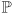(A) between 0 and 1 to the set A. This represents the probability that the random outcome belongs to the set A, and can be thought of as something like a notion of “mass” for the set A.

For this to work, we need(Ω) to be 1 (since the probability of getting something in the sample space must be 1). Also, if A and B are disjoint subsets of Ω, then(A B) should be(A) +(B). From this it follows that if A1, . . ., An are all disjoint, then(A1 . . . An) is equal to(A1) + . . . +(An). Actually, it turns out to be important that this should be true not just for finite unions but even for countably infinite [III.11] ones as well. (Related to this point is the fact that one does not attempt to define(A) for every subset A of Ω but just for measurable subsets [III.55]. For our purposes, it is sufficient to regard(A) as given whenever A is a set we can actually define.)

A probability space is a sample space Ω together with a function, defined on all “sensible” subsets A of Ω, that satisfies the conditions mentioned in the previous two paragraphs. The functionitself is known as a probability measure or probability distribution. The term probability distribution is often preferred when we specifyconcretely.

3 Continuous Probability Distributions

There are three particularly important distributions defined on subsets of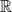, of which two will be discussed in this section. The first is the uniform distribution on the interval [0, 1]. We would like to capture the idea that “all points in [0, 1] are equally likely.” In view of the problems mentioned above, how should we do this?

A good way is to take seriously the “mass” metaphor. Although we cannot calculate the mass of an object by adding up the masses of all the infinitely small points that make up the object, we can assign to those points a density and integrate it. That is exactly what we shall do here. We assign a probability density of 1 to each point in the interval [0, 1]. Then we determine the probability of a subinterval, [1/3, ½ ] say, by calculating the integral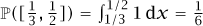. More generally, the probability associated with an interval [a, b] will just be its length ba. The probability of a union of disjoint intervals will then be the sum of the lengths of those intervals, and so on.

This “continuous” uniform distribution sometimes arises naturally from requirements of symmetry, just like its discrete counterpart. It can also arise as a limiting distribution. For instance, suppose that a hermit lives deep in a cave, away from any clocks or sources of natural light, and that each “day” he spends lasts for a random length of time between twenty-three and twenty-five hours. To start with, he will have some idea of what the time is, and be able to make statements such as, “I’m having lunch now, so it’s probably light outside,” but after a few weeks of this regime, he will no longer have any idea: any outside time will be just as likely as any other.

Now let us look at a rather more interesting density function, which depends on the choice of a positive constant λ. Consider the density function f(x) = λeλx, defined on the set of all nonnegative real numbers. To work out the probability associated with an interval [a, b], we now calculate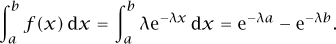The resulting probability distribution is called the exponential distribution with parameter λ. The exponential distribution is appropriate if we are modeling the time T of a spontaneous event, such as the time it takes for a radioactive nucleus to decay, or for the next spam email to arrive. The reason for this is based on the assumption of memorylessness: for example, if we know that the nucleus remains intact at time s, the probability that it will remain intact until a later time s + t is the same as the original probability that it would remain intact to time t. Let G(t) represent the probability that the nucleus remains intact up to time t. Then the probability that it remains intact up to time s + t given that it has remained intact up to time s is G(s + t)/G(s), so this has to equal G(t). Equivalently, G(s + t) = G(s)G(t). The only decreasing functions that have this property are exponential functions [III.25], that is, functions of the form G(t) = eλt for some positive λ. Since 1 − G(t) represents the probability that the nucleus decays before time t, this should equal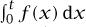, from which it is easy to deduce that f(x) = λeλx.

We shall come to the third, and most important, distribution below.

4 Random Variables, Mean, and Variance

Given a probability space, an event is defined to be a (sufficiently nice) subset of that space. For example, if the probability space is the interval [0, 1] with the uniform distribution, then the interval [½, 1] is an event: it represents a randomly chosen number between 0 and 1 turning out to be at least ½ . It is often useful to think not just about random events, but also about random numbers associated with a probability space. For example, let us look once again at a sequence of tosses of a biased coin that has probability p of coming up heads. The natural sample space associated with this experiment is the set Ω of all sequences ω of 0s and 1s. Earlier, we showed that the probability of obtaining k heads is, and we described that as a distribution on the sample space {0, 1, 2, . . ., n}. However, it is in many ways more natural, and often far more convenient, to regard the original set Ω as the sample space and to define a function X from Ω toto represent the number of heads: that is, X(ω) is the number of 1s in the sequence ω. We then write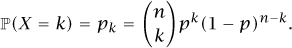A function like this is called a random variable. If X is a random variable and it takes values in a set Y, then the distribution of X is the function P defined on subsets of Y by the formula P(A) =(XA) =({ω ∈ Ω : X(ω) ∈ A}). It is not hard to see that P is indeed a probability distribution on Y.

For many purposes, it is enough to know the distribution of a random variable. However, the notion of a random variable defined on a sample space captures our intuition of a random quantity, and it allows us to ask further questions. For example, if we were to ask for the probability that there were k heads given that the first and last tosses had the same outcome, then the distribution of X would not provide the answer, whereas our richer model of regarding X as a function defined on sequences would do so. Furthermore, we can talk of independent random variables, X1, . . ., Xn say, meaning that the subset of Ω where Xi(ω) Ai for all i has probability given by the product(X1 A1) × . . . ×(Xn An) for all possible sets of values Ai.

Associated with a random variable X are two important numbers that begin to characterize it, called the mean or expectation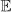(X) and the variance var(X). Both these numbers are determined by the distribution of X. If X takes integer values, with distribution(X = k) = pk, then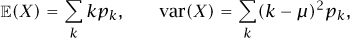where μ =(X). The mean tells us how big X is on average. The variance, or more precisely its square root, the standard deviation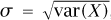, tells us how far away X lies, typically, from its mean. It is not hard to derive the following useful alternative formula for the variance: var(X) =(X2) –(X)2. To understand the meaning of the variance, consider the following situation. Suppose that one hundred people take an exam and you are told that their average mark is 75%. This gives you some useful information, but by no means a complete picture of how the marks are distributed. For example, perhaps the exam consisted of four questions of which three were very easy and one almost impossible, so that all the marks were clustered around 75%. Or perhaps about fifty people got full marks and fifty got around half marks. To model this situation let the sample space Ω consist of the hundred people and let the probability distribution be the uniform distribution. Given a random personω, let X(ω) be that person’s mark. Then in the first situation, the variance will be small, since almost everybody’s mark is close to the mean of 75%; whereas in the second it is close to 252 = 625, since almost everybody’s mark was about 25 away from the mean. Thus, the variance helps us to understand the difference between the two situations.

As we discussed at the start of this article, it is known from experience that the “expected” number of heads in a sequence of n tosses of a fair coin is around ½n, in the sense that the proportion is usually close to ½. It is not hard to work out that, if X models the number of heads in n tosses, that is, if X is binomially distributed with parameters n and ½, then(X) = ½n. The variance of X is ¼n, so the natural distance scale with which to measure the spread of the distribution is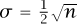. This allows us to see that X/n is close to ½ with probability close to 1 for large n, in accordance with experience.

More generally, if X1, X2, . . ., Xn are independent random variables, then var(X1 + . . . + Xn) = var(X1) + . . . + var(Xn). It follows that if all the Xi have the same distribution with mean μ and variance σ2, then the variance of the sample average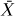= n1 (X1 + . . . + Xn) is n2(2) = σ2/n, which tends to zero as n tends to infinity. This observation can be used to prove that, for any ε > 0, the probability that |μ| is greater than ε tends to zero as n tends to infinity. Thus, the sample average “converges in probability” to the mean μ.

This result is called the weak law of large numbers. The argument sketched above implicitly assumes that the random variables have finite variance, but this assumption turns out not to be necessary. There is also a strong law of large numbers, which states that, with probability 1, the sample average of the first n variables converges to μ as n tends to infinity. As its name suggests, the strong law is stronger than the weak law, in the sense that the weak law can be deduced from the strong law. Notice that these laws make long-term predictions of a statistical kind about the real events that we have chosen to model using probability theory. Moreover, these predictions can be checked experimentally, and the experimental evidence confirms them. This provides a convincing scientific justification for our models.

5 The Normal Distribution and the Central Limit Theorem

As we have seen, for the binomial distribution with parameters n and p, the probability pk is given by the formula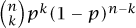. If n is large and you plot the points (k,pk) on a graph, then you will notice that they lie in a bell-shaped curve that has a sharp peak around the mean np. The width of the tall part of the curve has order of magnitude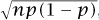, the standard deviation of the distribution. Let us assume for simplicity that np is an integer, and define a new probability distribution qk by qk = pk+np. The points (k, qk) peak at k = 0. If you now rescale the graph, compressing horizontally by a factor ofand expanding vertically by the same factor, then the points will all lie close to the graph of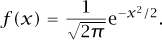This is the density function of a famous distribution known as the standard normal distribution on. It is also often called the Gaussian distribution.

To put this differently, if you toss a biased coin a large number of times, then the number of heads, minus its mean and divided by its standard deviation, is close to a standard normal random variable.

The function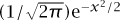occurs in a huge variety of mathematical contexts, from probability theory to fourier analysis [III.27] to quantum mechanics. Why should this be? The answer, as it is for many such questions, is that there are properties that this function has that are shared by no other function.

One such property is rotational invariance. Suppose once again that we are throwing a dart at a dartboard and aiming for the bull’s-eye. We could model this as the result of adding two independent normal distributions at right angles to each other: one for the x-coordinate and one for the y-coordinate (each having mean 0 and variance 1, say). If we do this, then the twodimensional “density function” is given by the formula (1/2π)ex2/2e−y2/2, which can conveniently be written as (1/2π)er2/2, where r denotes the length of (x, y). In other words, the density function depends only on the distance from the origin. (This is why it is called “rotationally invariant.”) This very appealing property holds in more dimensions as well. And it turns out to be quite easy to check that (1/2π)e–r2/2 is the only such function: more precisely, it is the only rotationinvariant density function that makes the coordinates x and y into independent random variables of variance 1. Thus, the normal distribution has a very special symmetry property.

Properties like this go some way toward explaining the ubiquity of the normal distribution in mathematics. However, the normal distribution has an even more remarkable property, which leads to its appearance wherever mathematics is used to model disorder in the real world. The central limit theorem states that, for any sequence of independent and identically distributed random variables X1, X2, . . . (with finite mean μ and nonzero finite variance σ2), we have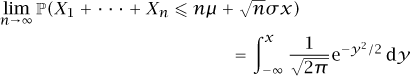for every real number x. The expected value of X1 + . . . + Xn is and its standard deviation is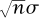, so another way of thinking about this is to let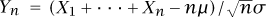. This rescales X1 + . . . + Xn to have mean 0 and variance 1, and the probability becomes the probability that Ynx. Thus, whatever distribution we start with, the limiting distribution of the sum of many independent copies is normal (after appropriate rescaling). Many natural processes can realistically be modeled as accumulations of small independent random effects, and this is why many distributions that one observes, such as the distribution of heights of adults in a given town, have a familiar bell-shaped curve.

A useful application of the central limit theorem is to simplify what look like impossibly complicated calculations. For example, when the parameter n is large, the calculation of binomial probabilities becomes prohibitively complicated. But if X is a binomial random variable, with parameters n and ½, for instance, then we can write X as a sum Y1 + . . . + Yn, where Y1, . . ., Yn are independent Bernoulli random variables with parameter ½ . Then, by the central limit theorem, lim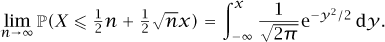Copyright © 2010 by Princeton University Press

Related Articles

Full text Article probability distribution
The Encyclopedia of Ecology and Environmental Management, Blackwell Science

A theoretical or observed distribution of probabilities or frequencies. Theoretical distributions use a priori probabilities, while observed...

Full text Article probability distribution
Focal Dictionary of Telecommunications, Focal Press

The spread of probability of occurrence. Examples are binomial distribution , Poisson distribution , normal distribution , exponential...

Full text Article distribution, probability
The Penguin Dictionary of Psychology

Similar to frequency distribution except that instead of pairing each class or category with the frequency with which it occurs it is paired...

See more from Credo